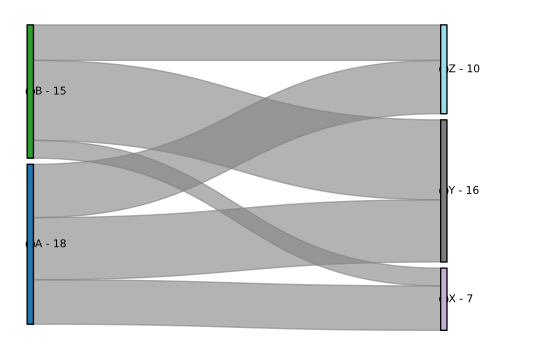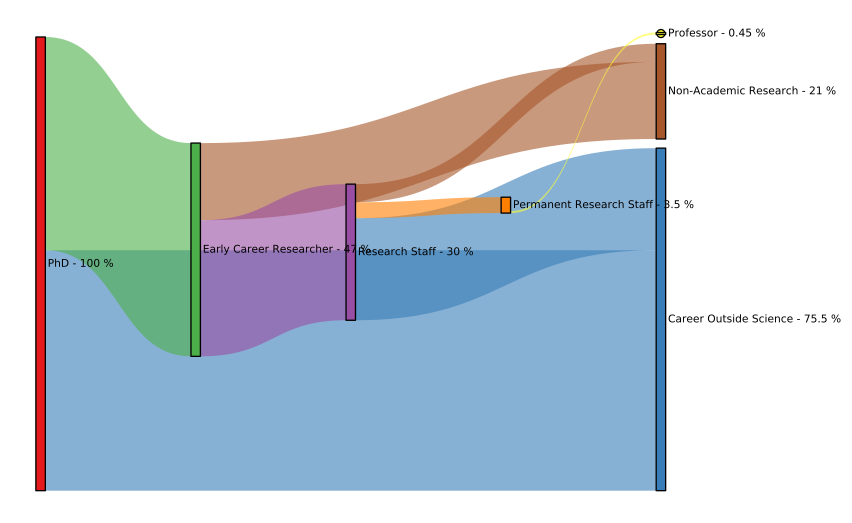# Sankey¶

#### Title: Sankey Element¶

Dependencies Bokeh

Backends Bokeh, Matplotlib

In :
import holoviews as hv
from holoviews import dim, opts

hv.extension('matplotlib')
hv.output(fig='svg')Sankey elements represent flows and their quantities in proportion to one another. The data of a Sankey element defines a directed, acyclic graph, making it a specialized subclass of the Graph element. The width of the lines in a Sankey diagram represent the magnitudes of each edge. Both the edges and nodes can be defined through any valid tabular format including pandas dataframes, dictionaries/tuples of columns, NumPy arrays and lists of tuples.

The easiest way to define a Sankey element is to define a list of edges and their associated quantities:

In :
hv.Sankey([
('A', 'X', 5),
('A', 'Y', 7),
('A', 'Z', 6),
('B', 'X', 2),
('B', 'Y', 9),
('B', 'Z', 4)]
).opts(fig_size=200)

Out:Above the node labels are generated automatically from the supplied edges, however, frequently the edges are expressed as integer node indexes and labels are provided separately. We can explicitly define the set of nodes as a Dataset of indexes and labels as key and value dimensions respectively. We can also use the edge_color style option to define a style mapping to a dimension and adjust the label_position from "right" to "left".

Here we will plot a simple dataset of the career paths of UK PhD students source as described in a 2010 Royal Society policy report entitled “The Scientific Century: securing our future prosperity”. We define the nodes enumerated by their integer index and the percentages flowing between each career stage. Finally we define a Dimension with units for the values and color by the target node which we label "To".

In :
nodes = ["PhD", "Career Outside Science",  "Early Career Researcher", "Research Staff",
"Permanent Research Staff",  "Professor",  "Non-Academic Research"]
nodes = hv.Dataset(enumerate(nodes), 'index', 'label')
edges = [
(0, 1, 53), (0, 2, 47), (2, 6, 17), (2, 3, 30), (3, 1, 22.5), (3, 4, 3.5), (3, 6, 4.), (4, 5, 0.45)
]

value_dim = hv.Dimension('Percentage', unit='%')
hv.Sankey((edges, nodes), ['From', 'To'], vdims=value_dim).opts(
opts.Sankey(cmap='Set1', labels='label', label_position='right', fig_size=300,
edge_color=dim('To').str(), node_color=dim('index').str()))

Out: